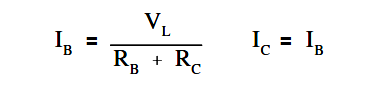An important property of a three-phase balanced system is that the phasor sum of the three line or phase voltages is zero, and the phasor sum of the three line or phase currents is zero. When the three load impedances are not equal to one another, the phasor sums and the neutral current (In) are not zero, and the load is, therefore, unbalanced. The imbalance occurs when an open or short circuit appears at the load.

If a three-phase system has an unbalanced load and an unbalanced power source, the methods of fixing the system are complex. Therefore, we will only consider an unbalanced load with a balanced power source.

Example:

A 3φ balanced system, as shown in Figure 16a, contains a wye load. The line-to- line voltage is 240V, and the resistance is 40 Ω in each branch.Figure 16 : 3φ Unbalanced Load

Find line current and neutral current for the following load conditions.

2. open circuit phase A (Figure 16b)
3. short circuit in phase A (Figure 16c)IL = 138.7/40

IL = 3.5 amps

IN = 0

Solution : Answer for open circuit phase A

2. Current flow in lines B and C becomes the resultant of the loads in B and C connected in series.IB = 240/40+40

IB = 3 amps

so, I= 3 amps

I= IB + I= 6 amps

3. Answer for short circuit in phase AIB = 240/40

IB = 6 amps

so IC = 6 amps

The current in Phase A is equal to the neutral line current, IA = IN

Therefore, IN is the phasor sum of IB = IC

IN = √3 IB

IN = 1.73 x 6

IN = 10.4 amps

In a fault condition, the neutral connection in a wye-connected load will carry more current than the phase under a balanced load. Unbalanced three-phase circuits are indicated by abnormally high currents in one or more of the phases. This may cause damage to equipment if the imbalance is allowed to continue.